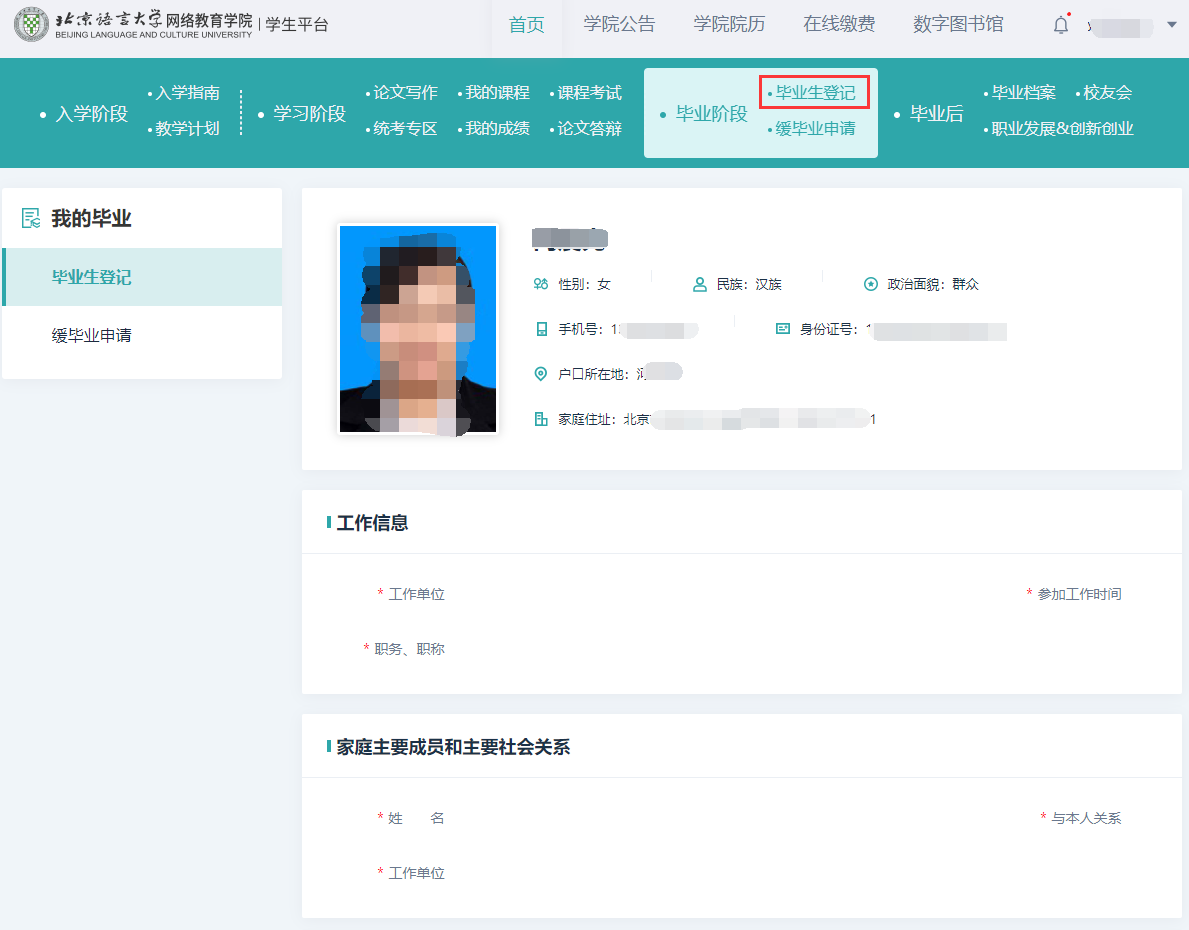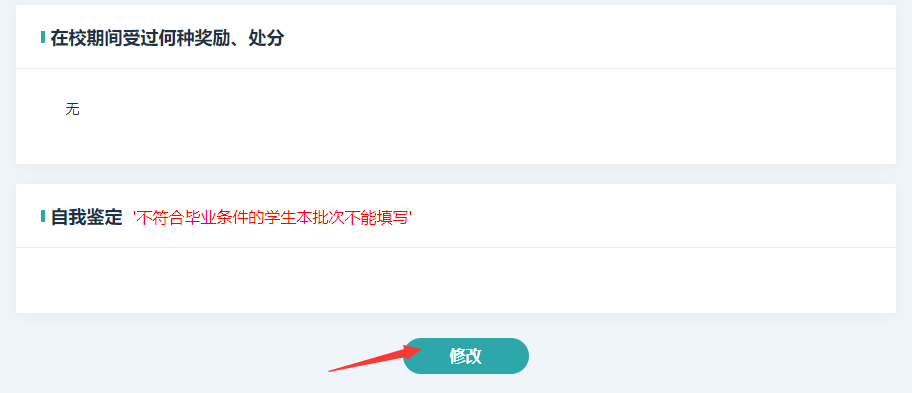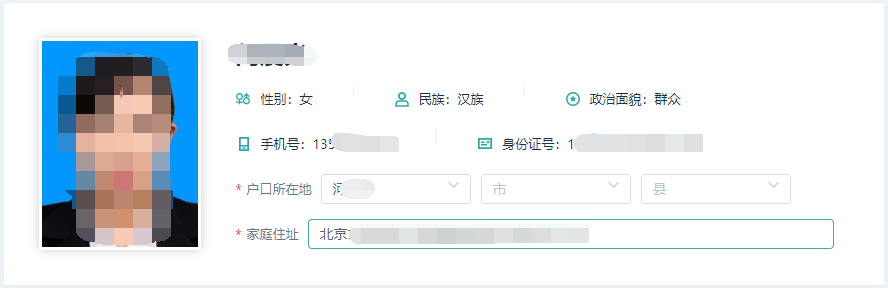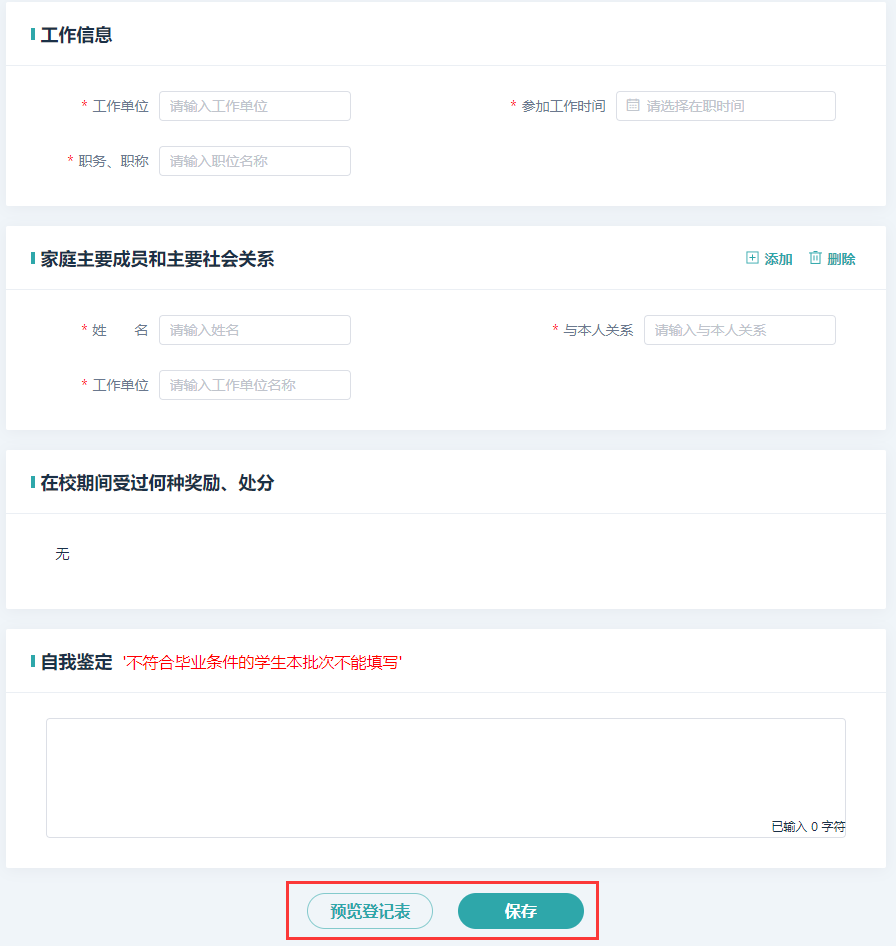## 关于202303毕业批次学生填写毕业生登记表的通知

2023-03-07 15:25:14

1、功能位置

2、填写规则

3、操作流程及详细说明点击【毕业生登记】按钮，会显示学生基本信息及需要填写的信息。如果有的信息平台中没有，那么学生基本信息上会显示“无”。学生基本信息取自学生入学时填写的报名表信息，如信息有误，请及时联系学习中心申请信息修改，办理信息修改的学生毕业顺延到下一批次。

核对学生基本信息后，点击下方【修改】按钮，开始填写。1．点击选择户口所在地的省、市、县。

2. 填写家庭住址。

3．如无工作单位和职务、职称，填“无”，不允许为空。

4. 点击选择参加工作的时间

家庭主要人员和主要社会关系

1. 姓名：允许最长录入16个汉字

2. 与本人关系：允许最长录入10个汉字

3. 工作单位：允许最长录入21个汉字

4. 最多录入4个家庭成员，如果无工作单位，填“无”，不允许为空。

在校期间受过何等奖励、处分

1.自动提取平台数据，不用填写。

自我鉴定预览显示要求同版模版。预览格式如下：

 姓名 粱** 性别 男 民族 汉族 政治面貌 群众 户口所在地 北京市海淀区 证件号码 110xxxxxxxxxxxxxxx 工作单位 取平台的“工作单位” 参加工作 时间 取平台数据 职务、职称 取平台的“职业” 家庭住址 取平台的“通讯地址” 联系电话 取平台的“移动电话” 家庭主要成员和主要社会关系 姓名 与本人 关系 工作单位 粱** 父子 北京语言大学 王** 母子 弘成教育 在校期间受过何种奖励、处分 取平台数据

 X X X X X X X X X X X X X X X X X X X X X X X X X X X X X X X X X X X X X X X X X X X X X X X X X X X X X X X X X X X X X X X X X X X X X X X X X X X X X X X X X X X X X X X X X X X X X X X X X X X X X X X X X X X X X X X X X X X X X X X X X X X X X X X X X X X X X X X X X X X X X X X X X X X X X X X X X X X X X X X X X X X X X X X X X X X X X X X X X X。                                                     本 人 签 名： 粱**                                                        2022年 7月 X X 日

北京语言大学网络教育学院

2023年3月7日